Ex 6.3

Chapter 6 Class 12 Application of Derivatives
Serial order wise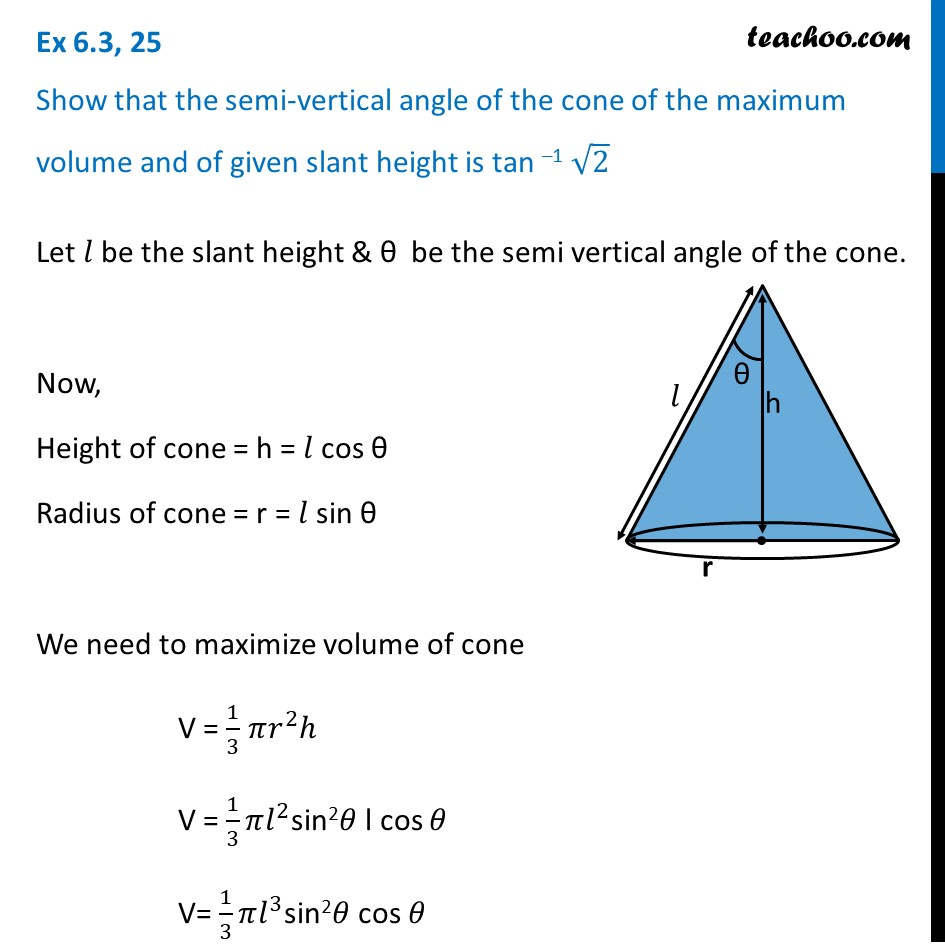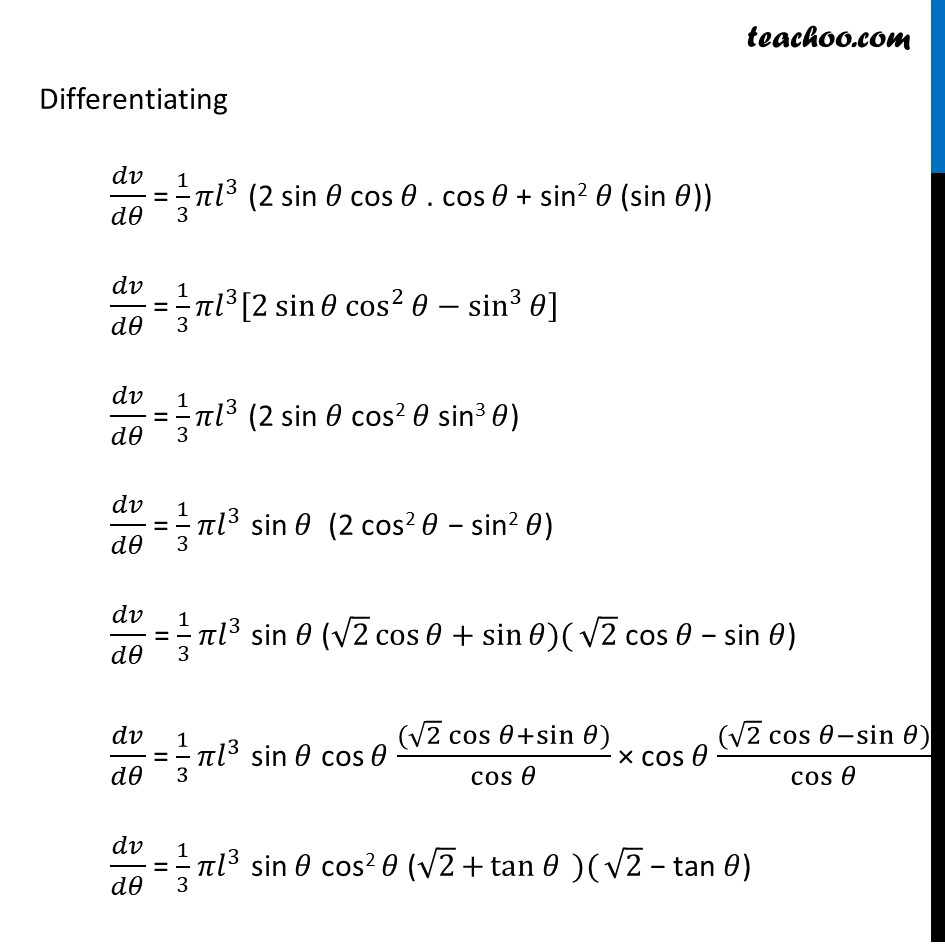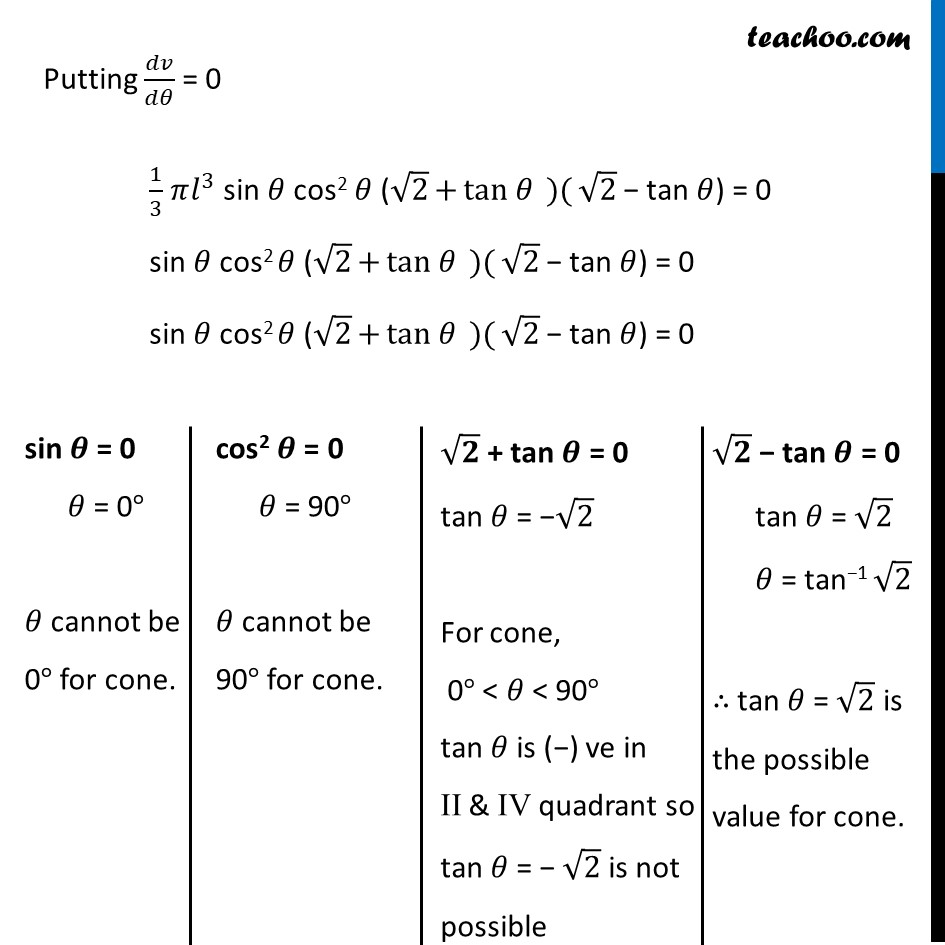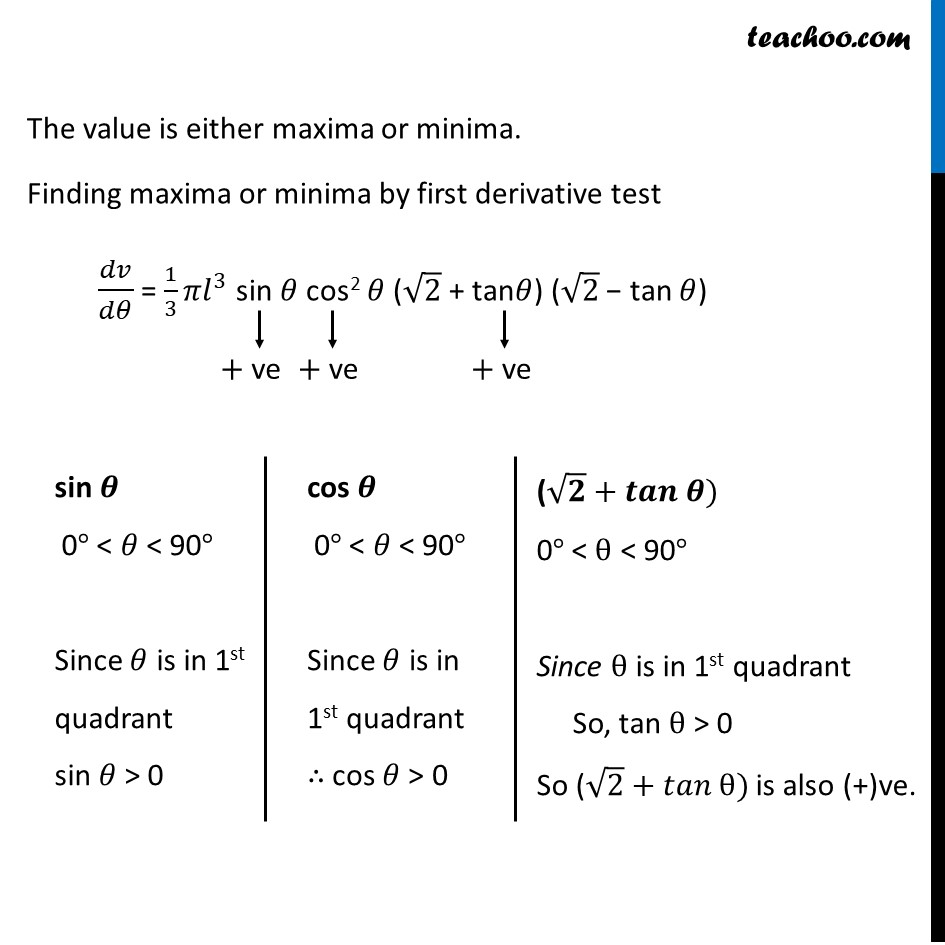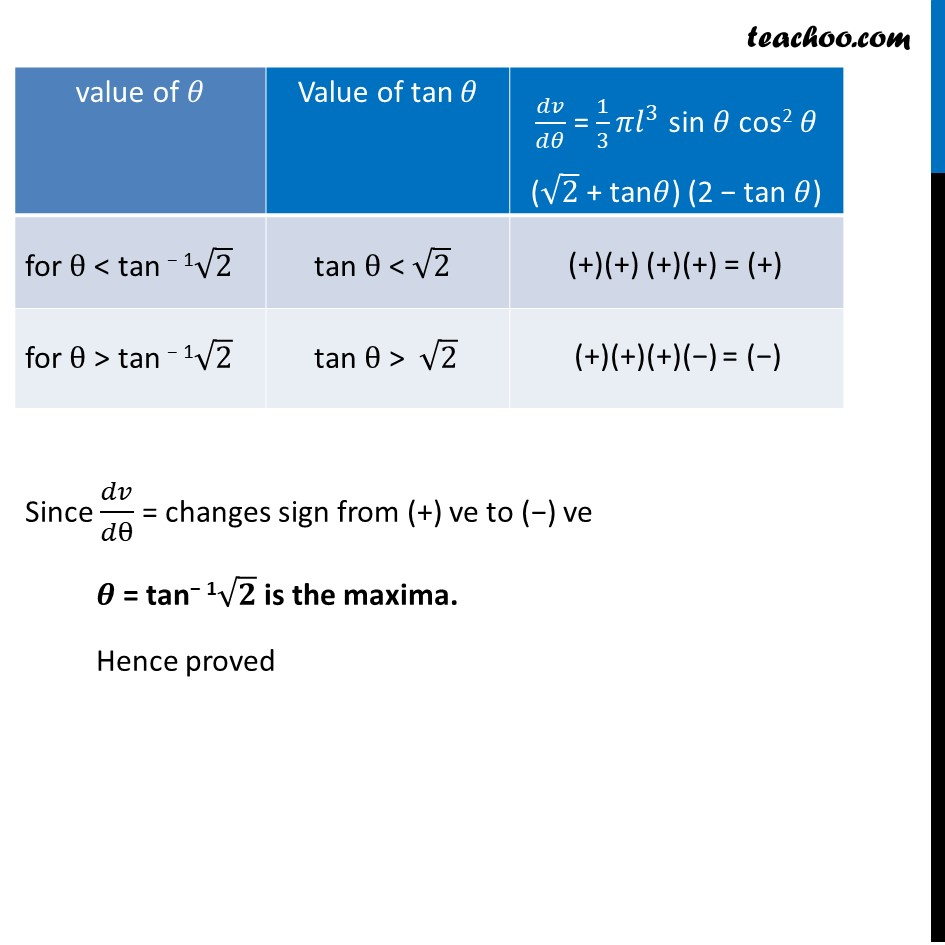Learn in your speed, with individual attention - Teachoo Maths 1-on-1 Class

### Transcript

Ex 6.3, 25 Show that the semi-vertical angle of the cone of the maximum volume and of given slant height is tan –1 √2Let 𝑙 be the slant height & θ be the semi vertical angle of the cone. Now, Height of cone = h = 𝑙 cos θ Radius of cone = r = 𝑙 sin θ We need to maximize volume of cone V = 1/3 𝜋𝑟^2 ℎ V = 1/3 𝜋𝑙^2sin2𝜃 l cos 𝜃 V= 1/3 𝜋𝑙^3sin2𝜃 cos 𝜃 Differentiating 𝑑𝑣/𝑑𝜃 = 1/3 𝜋𝑙^3 (2 sin 𝜃 cos 𝜃 . cos 𝜃 + sin2 𝜃 (sin 𝜃)) 𝑑𝑣/𝑑𝜃 = 1/3 𝜋𝑙^3 [2 sin⁡𝜃 cos^2⁡𝜃−sin^3⁡𝜃 ] 𝑑𝑣/𝑑𝜃 = 1/3 𝜋𝑙^3 (2 sin 𝜃 cos2 𝜃 sin3 𝜃) 𝑑𝑣/𝑑𝜃 = 1/3 𝜋𝑙^3 sin 𝜃 (2 cos2 𝜃 − sin2 𝜃) 𝑑𝑣/𝑑𝜃 = 1/3 𝜋𝑙^3 sin 𝜃 (√2 cos⁡〖𝜃+sin⁡〖𝜃)(〗 〗 √2 cos 𝜃 − sin 𝜃) 𝑑𝑣/𝑑𝜃 = 1/3 𝜋𝑙^3 sin 𝜃 cos 𝜃 ((√2 cos⁡〖𝜃+sin⁡𝜃 〗))/cos⁡𝜃 × cos 𝜃 ((√2 cos⁡〖𝜃−sin⁡𝜃 〗))/cos⁡𝜃 𝑑𝑣/𝑑𝜃 = 1/3 𝜋𝑙^3 sin 𝜃 cos2 𝜃 (√2⁡〖+〖tan 𝜃〗⁡〖 )(〗 〗 √2 − tan 𝜃) Putting 𝑑𝑣/𝑑𝜃 = 0 1/3 𝜋𝑙^3 sin 𝜃 cos2 𝜃 (√2⁡〖+〖tan 𝜃〗⁡〖 )(〗 〗 √2 − tan 𝜃) = 0 sin 𝜃 cos2 𝜃 (√2⁡〖+〖tan 𝜃〗⁡〖 )(〗 〗 √2 − tan 𝜃) = 0 sin 𝜃 cos2 𝜃 (√2⁡〖+〖tan 𝜃〗⁡〖 )(〗 〗 √2 − tan 𝜃) = 0 sin 𝜽 = 0 𝜃 = 0° 𝜃 cannot be 0° for cone. cos2 𝜽 = 0 𝜃 = 90° 𝜃 cannot be 90° for cone. √𝟐 + tan 𝜽 = 0 tan 𝜃 = −√2 For cone, 0° < 𝜃 < 90° tan 𝜃 is (−) ve in II & IV quadrant so tan 𝜃 = − √2 is not possible √𝟐 − tan 𝜽 = 0 tan 𝜃 = √2 𝜃 = tan−1 √2 ∴ tan 𝜃 = √2 is the possible value for cone. sin 𝜽 0° < 𝜃 < 90° Since 𝜃 is in 1st quadrant sin 𝜃 > 0 cos 𝜽 0° < 𝜃 < 90° Since 𝜃 is in 1st quadrant ∴ cos 𝜃 > 0 (√𝟐+𝒕𝒂𝒏 𝜽) 0° < θ < 90° Since θ is in 1st quadrant So, tan θ > 0 So (√2+𝑡𝑎𝑛 θ) is also (+)ve. Since 𝑑𝑣/𝑑θ = changes sign from (+) ve to (−) ve 𝜽 = tan− 1√𝟐 is the maxima. Hence proved